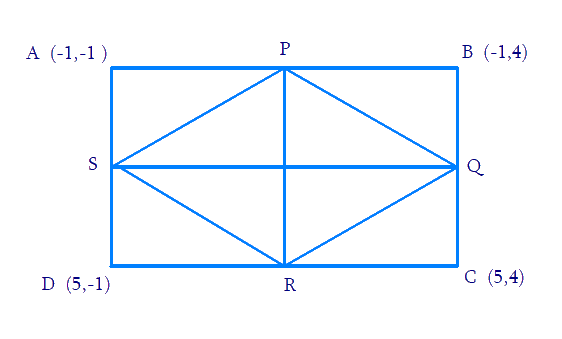# Ex.7.4 Q8 Coordinate Geometry Solution - NCERT Maths Class 10

Go back to  'Ex.7.4'

## Question

$$ABCD$$ is a rectangle formed by the points $$A \;(- 1, - 1)$$, $$B\; (- 1, 4)$$, $$C \;(5, 4)$$ and $$D\; (5, - 1)$$. $$P$$, $$Q$$, $$R$$ and $$S$$ are the mid-points of $$AB$$, $$BC$$, $$CD$$, and $$DA$$ respectively. Is the quadrilateral $$PQRS$$ is a square? A rectangle? Or a rhombus? Justify your answer.

Video Solution
Coordinate Geometry
Ex 7.4 | Question 8

## Text Solution

Reasoning:

The distance between the two points can be measured using the Distance Formula which is given by:

Distance Formula

\begin{align}= \sqrt {{{\left( {{x_1} - {x_2}} \right)}^2} + {{\left( {{y_1} - {y_2}} \right)}^2}} .\end{align}

What is known?

The $$x$$ and $$y$$ coordinates of the vertices of the rectangle.

What is unknown?

To find whether quadrilateral $$PQRS$$ is a square? A rectangle? Or a rhombus?

Steps:

From the figure below,$$P$$ is the mid-point of side $$AB$$. The co-ordinates of $$P$$ can be calculated using the Mid-Point Formula as

\begin{align}M = \left( {\frac{{{x_1} + {x_2}}}{2},\frac{{{y_1} + {y_2}}}{2}} \right)\end{align}

Therefore, the coordinates of $$P$$ are

\begin{align}\left[ {\frac{{ - 1 - 1}}{2},\,\frac{{ - 1 + 4}}{2}} \right) = \left( { - 1,\,\,\frac{3}{2}} \right] \end{align}

Similarly, the coordinates of $$Q$$, $$R$$ and $$S$$ are calculated using the Mid-Point Formula as :

$$(2,4),\left( {5,\frac{3}{2}} \right)\,{\text{and}}\,(2, - 1)$$ respectively.

We know that the distance between the two points is given by the Distance Formula,

\begin{align} & \sqrt {{{\left( {{x_1} - {x_2}} \right)}^2} + {{\left( {{y_1} - {y_2}} \right)}^2}} \ldots (1) \end{align}

Distance between two points $$P$$ and $$Q$$,

Length of $$PQ$$

\begin{align} &= \sqrt {{{( - 1 - 2)}^2} + {{\left( {\frac{3}{2} - 4} \right)}^2}} \\ &= \sqrt {9 + \frac{{25}}{4}} \\& = \sqrt {\frac{{61}}{4}} \end{align}

Distance between two points $$Q$$ and $$R$$,

Length of $$QR$$

\begin{align} &= \sqrt {{{(2 - 5)}^2} + {{\left( {4 - \frac{3}{2}} \right)}^2}} \\ &= \sqrt {9 + \frac{{25}}{4}} \\ &= \sqrt {\frac{{61}}{4}} \end{align}

Distance between two points $$R$$ and $$S$$,

Length of $$RS$$

\begin{align} &= \sqrt {{{(5 - 2)}^2} + {{\left( {\frac{3}{2} + 1} \right)}^2}} \\ &= \sqrt {9 + \frac{{25}}{4}} \\ &= \sqrt {\frac{{61}}{4}} \end{align}

Distance between two points $$S$$ and $$P$$,

Length of $$SP$$

\begin{align} &= \sqrt {{{(2 + 1)}^2} + {{\left( { - 1 - \frac{3}{2}} \right)}^2}} \\& = \sqrt {9 + \frac{{25}}{4}} \\ &= \sqrt {\frac{{61}}{4}}\end{align}

Distance between two points $$P$$ and $$R$$ which form the diagonal are ,

Length of $$PR$$

\begin{align} &= \sqrt {{{( - 1 - 5)}^2} + \left( {\frac{3}{2}} \right) - \frac{{{3^2}}}{2}} \\ &= 6\end{align}

Distance between two points $$Q$$ and $$S$$ which form the diagonal is calculated using the Distance Formula as,

Length of $$QS$$

\begin{align}{\text{ }}&= \,\sqrt {{{(2 - 2)}^2} + {{(4 + 1)}^2}} \\ &= 5\end{align}

It can be observed that all sides of the given quadrilateral are of the same measure. However, the diagonals are of different lengths. Therefore, $$PQRS$$ is a rhombus.

Learn from the best math teachers and top your exams

• Live one on one classroom and doubt clearing
• Practice worksheets in and after class for conceptual clarity
• Personalized curriculum to keep up with school